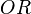# Odds facts for kids

Kids Encyclopedia Facts

In mathematics, odds are the chance that an event will happen compared to the chance that it will not happen. For example, if there is an equal chance that it will rain or that it will not rain, the odds of it raining are 1:1 (which is read as "1 to 1"). If it is twice as likely that it will not rain, then the odds of it raining are 1:2 (read as "1 to 2").

## Uses for odds

Odds are often used in gambling, especially in horse racing.

In statistics, when the odds are written as fractions, the ratio of the odds of two related experimental events is called the odds ratio. This is often written as$OR$ for short.

## Related pagesOdds Facts for Kids. Kiddle Encyclopedia.